Скачать презентацию Stacks Outline and Reading The Stack ADT

a2465cf07397d1ffe931a9d05e0586a6.ppt

• Количество слайдов: 20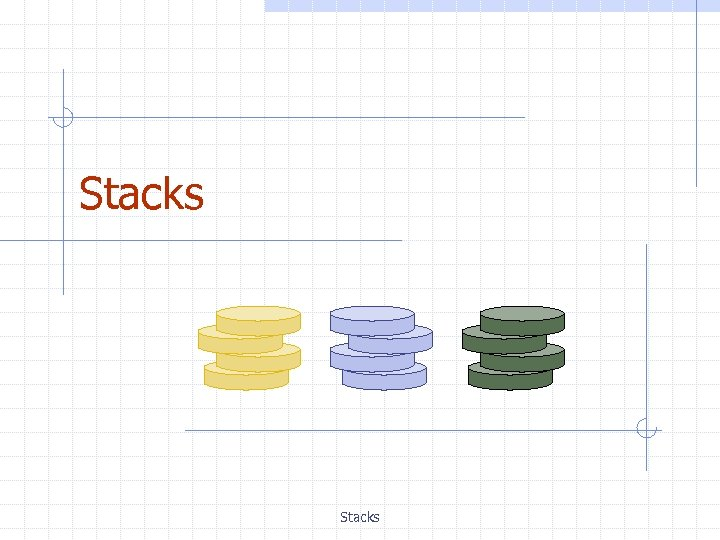Stacks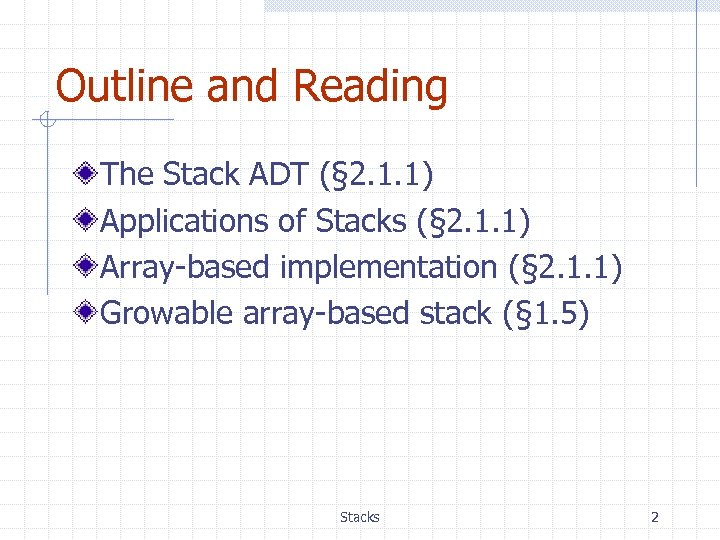Outline and Reading The Stack ADT (§ 2. 1. 1) Applications of Stacks (§ 2. 1. 1) Array-based implementation (§ 2. 1. 1) Growable array-based stack (§ 1. 5) Stacks 2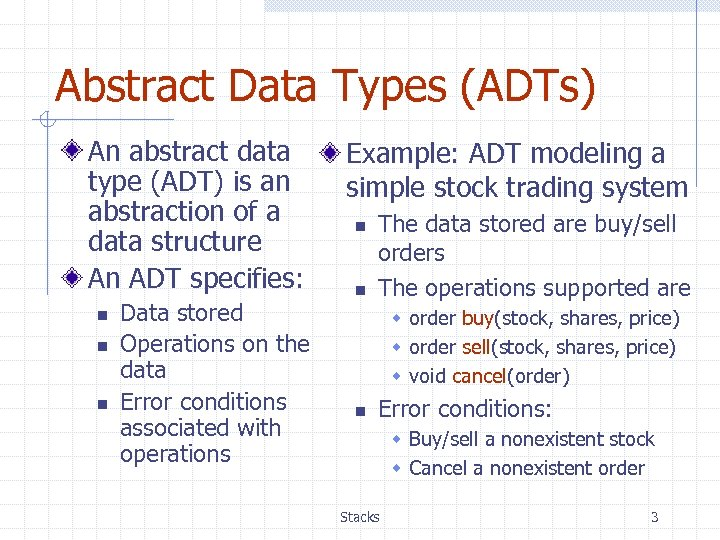Abstract Data Types (ADTs) An abstract data type (ADT) is an abstraction of a data structure An ADT specifies: n n n Data stored Operations on the data Error conditions associated with operations Example: ADT modeling a simple stock trading system n n The data stored are buy/sell orders The operations supported are w order buy(stock, shares, price) w order sell(stock, shares, price) w void cancel(order) n Error conditions: w Buy/sell a nonexistent stock w Cancel a nonexistent order Stacks 3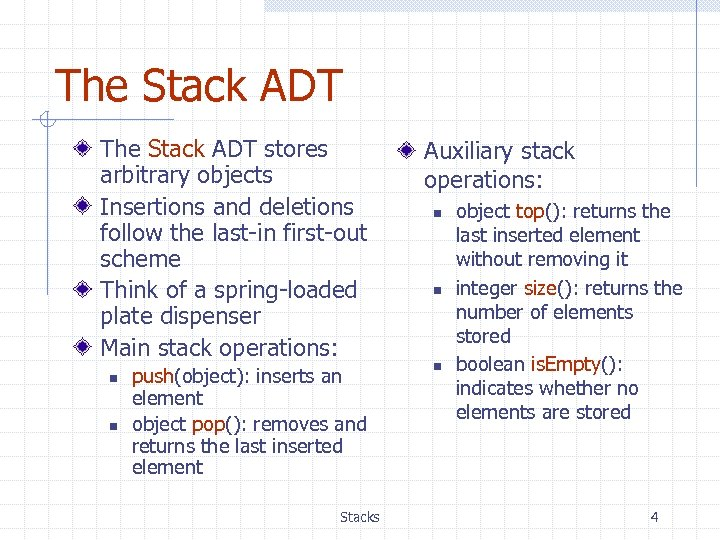The Stack ADT stores arbitrary objects Insertions and deletions follow the last-in first-out scheme Think of a spring-loaded plate dispenser Main stack operations: n n push(object): inserts an element object pop(): removes and returns the last inserted element Stacks Auxiliary stack operations: n n n object top(): returns the last inserted element without removing it integer size(): returns the number of elements stored boolean is. Empty(): indicates whether no elements are stored 4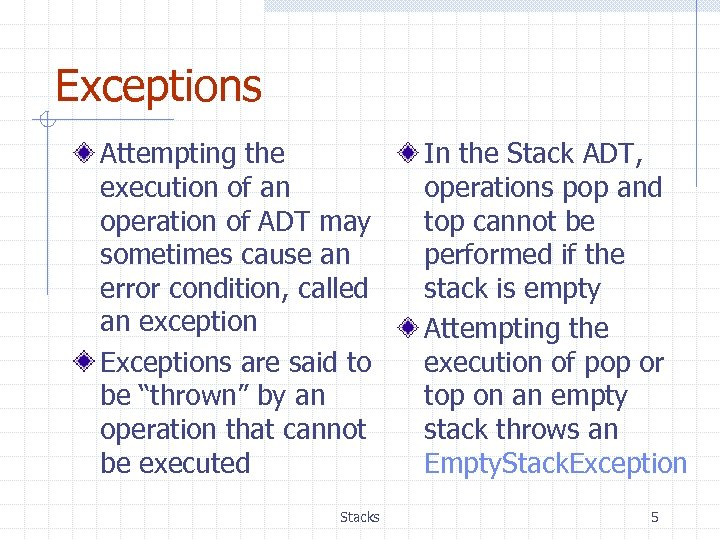Exceptions Attempting the execution of an operation of ADT may sometimes cause an error condition, called an exception Exceptions are said to be “thrown” by an operation that cannot be executed Stacks In the Stack ADT, operations pop and top cannot be performed if the stack is empty Attempting the execution of pop or top on an empty stack throws an Empty. Stack. Exception 5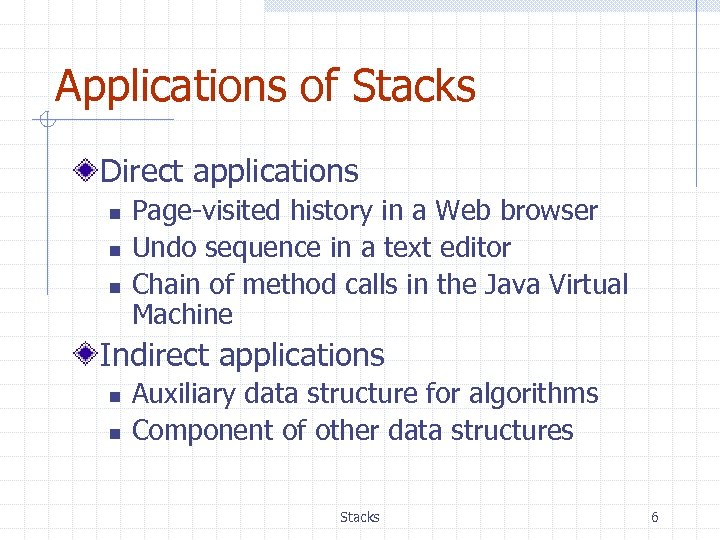Applications of Stacks Direct applications n n n Page-visited history in a Web browser Undo sequence in a text editor Chain of method calls in the Java Virtual Machine Indirect applications n n Auxiliary data structure for algorithms Component of other data structures Stacks 6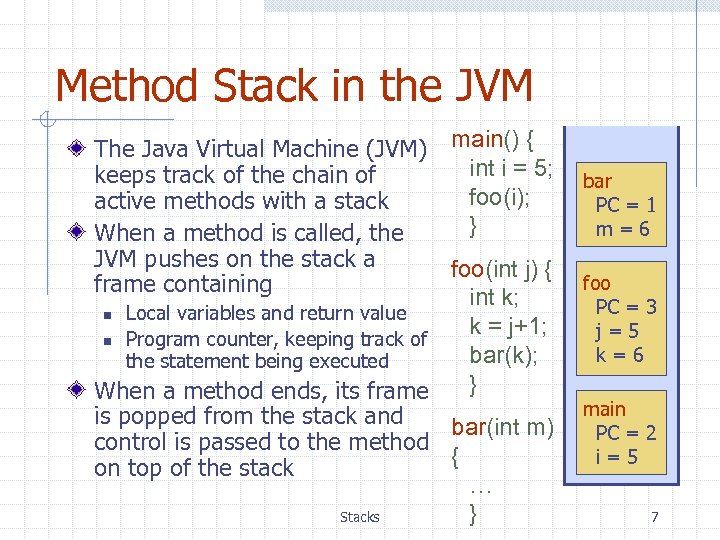Method Stack in the JVM The Java Virtual Machine (JVM) keeps track of the chain of active methods with a stack When a method is called, the JVM pushes on the stack a frame containing main() { int i = 5; foo(i); } foo(int j) { int k; n Local variables and return value k = j+1; n Program counter, keeping track of bar(k); the statement being executed } When a method ends, its frame is popped from the stack and bar(int m) control is passed to the method { on top of the stack … } Stacks bar PC = 1 m=6 foo PC = 3 j=5 k=6 main PC = 2 i=5 7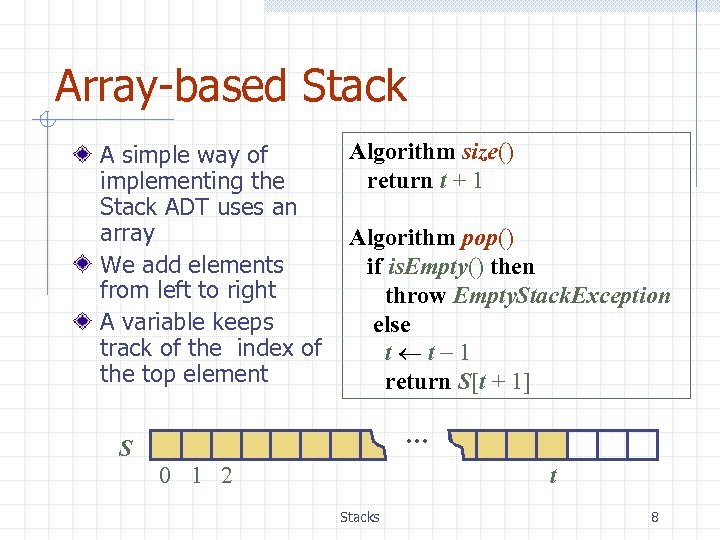Array-based Stack A simple way of implementing the Stack ADT uses an array We add elements from left to right A variable keeps track of the index of the top element Algorithm size() return t + 1 Algorithm pop() if is. Empty() then throw Empty. Stack. Exception else t t 1 return S[t + 1] … S 0 1 2 t Stacks 8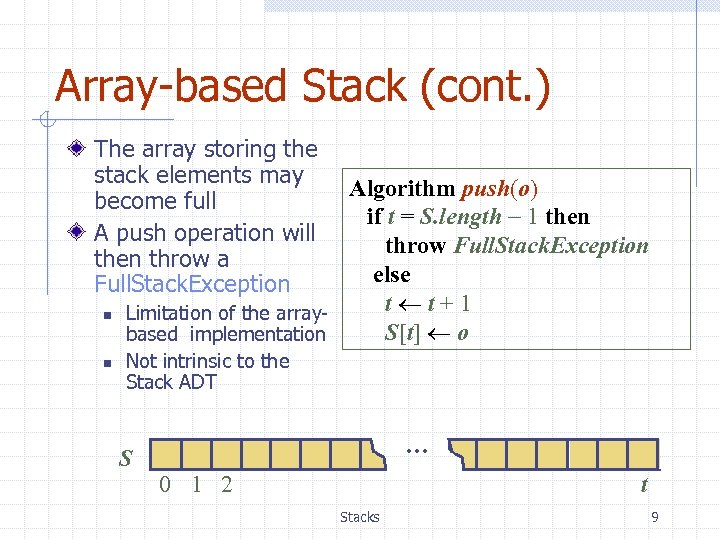Array-based Stack (cont. ) The array storing the stack elements may become full A push operation will then throw a Full. Stack. Exception n n Algorithm push(o) if t = S. length 1 then throw Full. Stack. Exception else t t+1 Limitation of the array. S[t] o based implementation Not intrinsic to the Stack ADT … S 0 1 2 t Stacks 9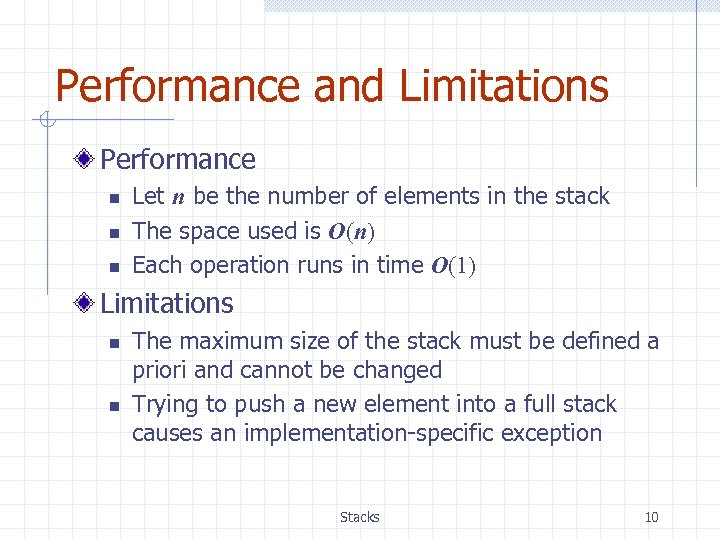Performance and Limitations Performance n n n Let n be the number of elements in the stack The space used is O(n) Each operation runs in time O(1) Limitations n n The maximum size of the stack must be defined a priori and cannot be changed Trying to push a new element into a full stack causes an implementation-specific exception Stacks 10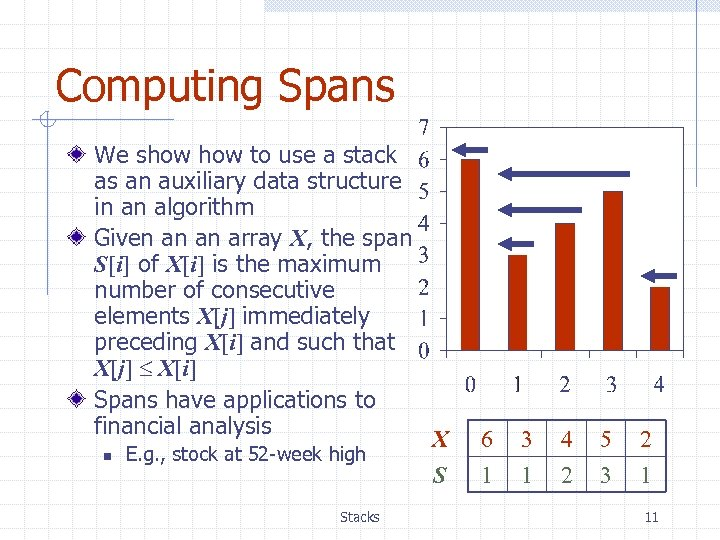Computing Spans We show to use a stack as an auxiliary data structure in an algorithm Given an an array X, the span S[i] of X[i] is the maximum number of consecutive elements X[j] immediately preceding X[i] and such that X[j] X[i] Spans have applications to financial analysis n E. g. , stock at 52 -week high Stacks X S 6 1 3 1 4 2 5 3 2 1 11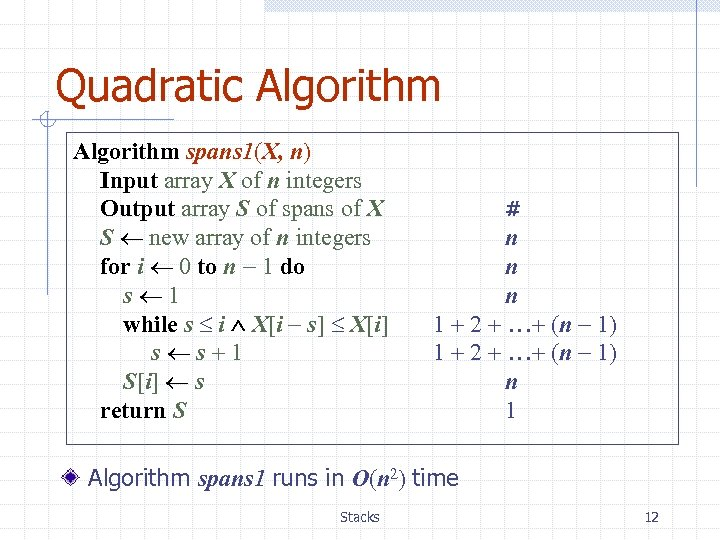Quadratic Algorithm spans 1(X, n) Input array X of n integers Output array S of spans of X S new array of n integers for i 0 to n 1 do s 1 while s i X[i s] X[i] s s+1 S[i] s return S # n n n 1 + 2 + …+ (n 1) n 1 Algorithm spans 1 runs in O(n 2) time Stacks 12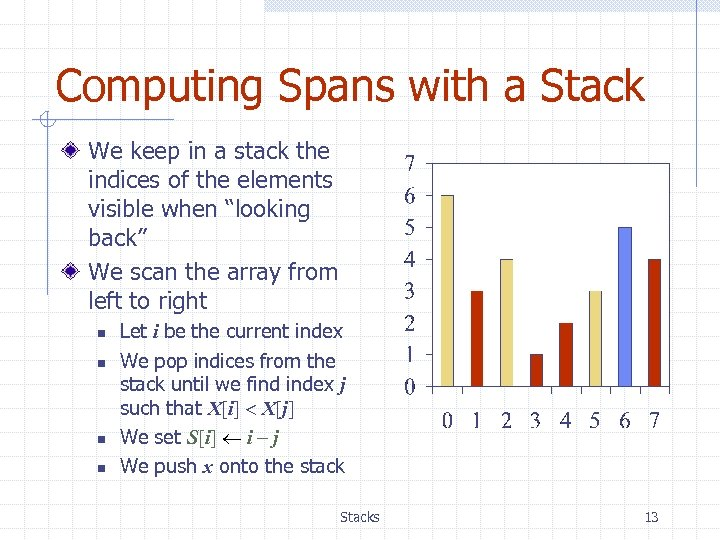Computing Spans with a Stack We keep in a stack the indices of the elements visible when “looking back” We scan the array from left to right n n Let i be the current index We pop indices from the stack until we find index j such that X[i] X[j] We set S[i] i j We push x onto the stack Stacks 13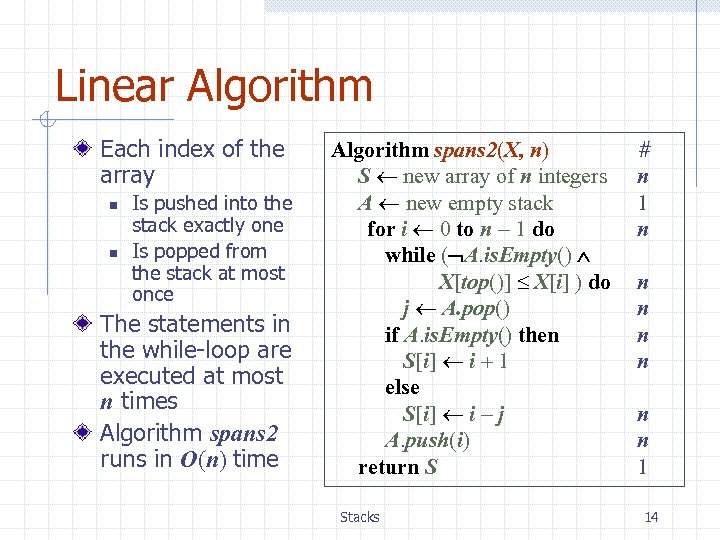Linear Algorithm Each index of the array n n Is pushed into the stack exactly one Is popped from the stack at most once The statements in the while-loop are executed at most n times Algorithm spans 2 runs in O(n) time Algorithm spans 2(X, n) S new array of n integers A new empty stack for i 0 to n 1 do while ( A. is. Empty() X[top()] X[i] ) do j A. pop() if A. is. Empty() then S[i] i + 1 else S[i] i j A. push(i) return S Stacks # n 1 n n n n 1 14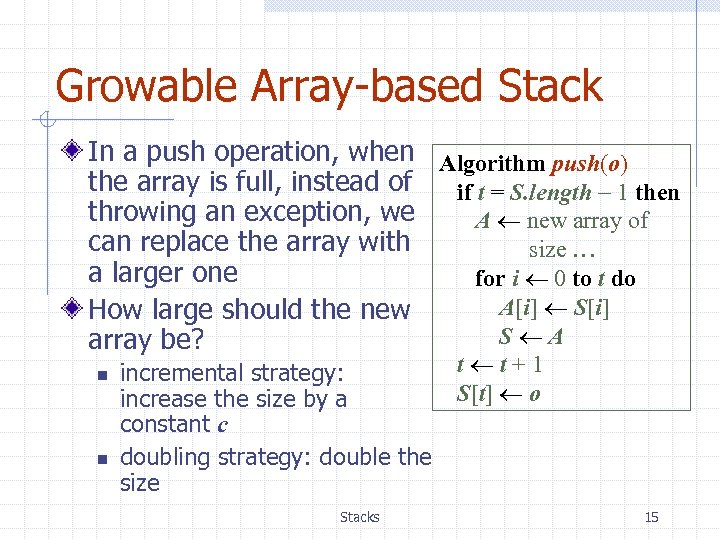Growable Array-based Stack In a push operation, when Algorithm push(o) the array is full, instead of if t = S. length 1 then throwing an exception, we A new array of can replace the array with size … a larger one for i 0 to t do A[i] S[i] How large should the new S A array be? n n incremental strategy: increase the size by a constant c doubling strategy: double the size Stacks t t+1 S[t] o 15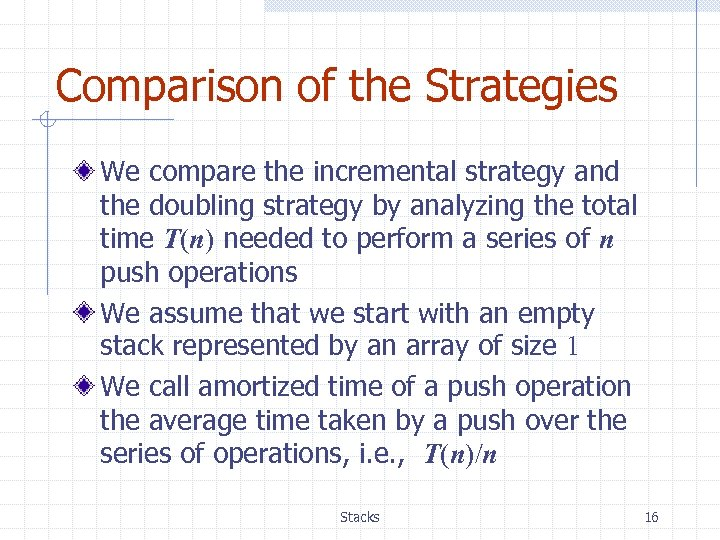Comparison of the Strategies We compare the incremental strategy and the doubling strategy by analyzing the total time T(n) needed to perform a series of n push operations We assume that we start with an empty stack represented by an array of size 1 We call amortized time of a push operation the average time taken by a push over the series of operations, i. e. , T(n)/n Stacks 16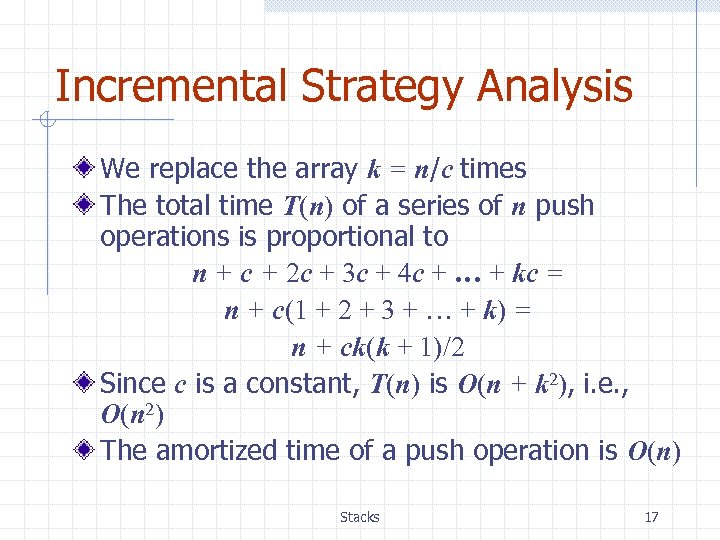Incremental Strategy Analysis We replace the array k = n/c times The total time T(n) of a series of n push operations is proportional to n + c + 2 c + 3 c + 4 c + … + kc = n + c(1 + 2 + 3 + … + k) = n + ck(k + 1)/2 Since c is a constant, T(n) is O(n + k 2), i. e. , O(n 2) The amortized time of a push operation is O(n) Stacks 17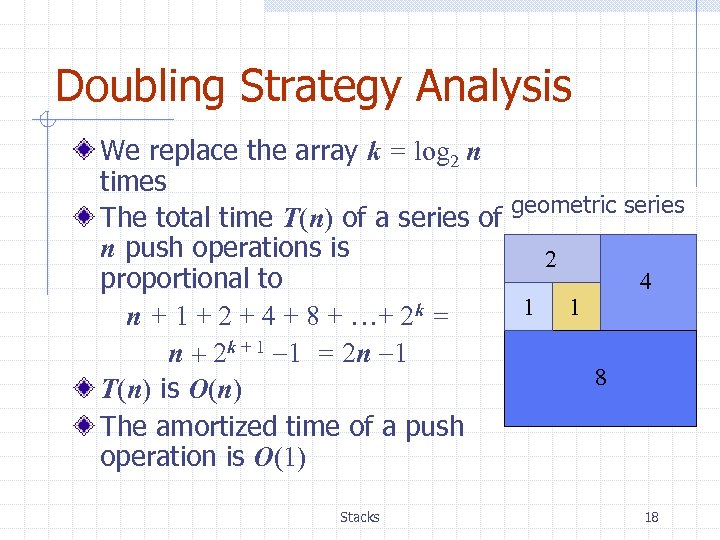Doubling Strategy Analysis We replace the array k = log 2 n times The total time T(n) of a series of geometric series n push operations is 2 proportional to 4 1 1 n + 1 + 2 + 4 + 8 + …+ 2 k = n + 2 k + 1 1 = 2 n 1 8 T(n) is O(n) The amortized time of a push operation is O(1) Stacks 18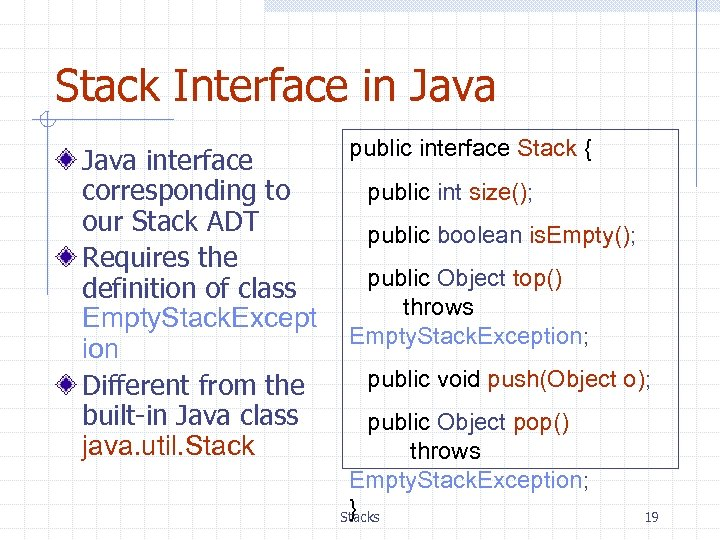Stack Interface in Java interface corresponding to our Stack ADT Requires the definition of class Empty. Stack. Except ion Different from the built-in Java class java. util. Stack public interface Stack { public int size(); public boolean is. Empty(); public Object top() throws Empty. Stack. Exception; public void push(Object o); public Object pop() throws Empty. Stack. Exception; } Stacks 19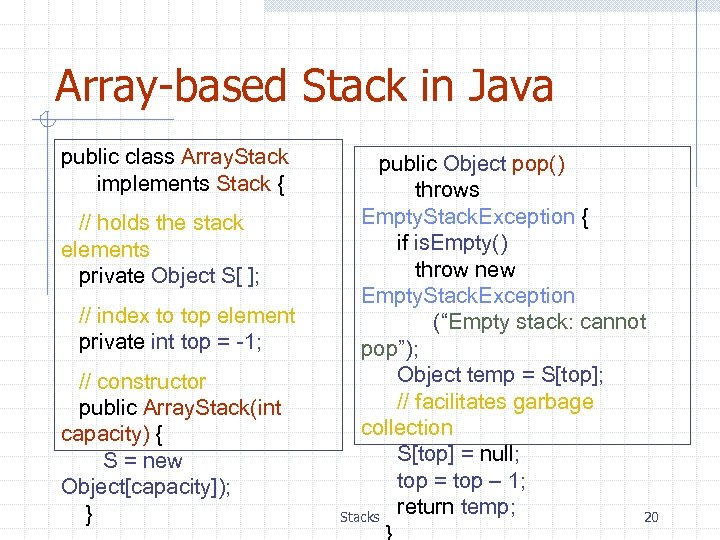Array-based Stack in Java public class Array. Stack implements Stack { // holds the stack elements private Object S[ ]; // index to top element private int top = -1; // constructor public Array. Stack(int capacity) { S = new Object[capacity]); } public Object pop() throws Empty. Stack. Exception { if is. Empty() throw new Empty. Stack. Exception (“Empty stack: cannot pop”); Object temp = S[top]; // facilitates garbage collection S[top] = null; top = top – 1; return temp; Stacks 20